# Dirichlet's condition

Dirichlet's condition is a mathematical condition used in the study of Fourier series. Specifically, it is a sufficient condition for the convergence of a Fourier series to the function it represents.

The condition is named after the German mathematician Johann Peter Gustav Lejeune Dirichlet, who first stated and proved it in the mid-19th century.

The condition states that if a function f(x) is periodic with period 2π and has a finite number of discontinuities in each period, and if its derivative is piecewise continuous in each period, then the Fourier series of f(x) converges to f(x) at every point of continuity of f(x) and converges to the average of the left and right limits of f(x) at every point of discontinuity.

In other words, Dirichlet's condition ensures that a Fourier series converges pointwise to the original function, except possibly at a finite number of points where the function is discontinuous.

Dirichlet's condition is a useful tool in the analysis of periodic functions and has applications in various fields such as signal processing, image analysis, and physics.

Dirichlet's condition: For a function f(x) defined in the [ - π , π ] if f(x) is

1. Single valued

2. Bounded

3. Piecewise continuous i.e has finite number of discontinuities

4. has finite maxima or minima

5. defined outside the interval [ - π , π ] then, f(x) repeats i.e

f(x+ 2π ) = f(x)

then the partial sum δp (x) of f(x) is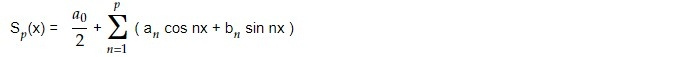converse to f(x) for p ∞ at the point of x where f(x) is continuous and converges to 1/2 [ f(x+0) + f(x-0)] for p ∞ at the point of x where f(x) is discontinuous.

Proof of Dirichlet's condition: For a function defined in interval [ -π , π], the partial sum is given by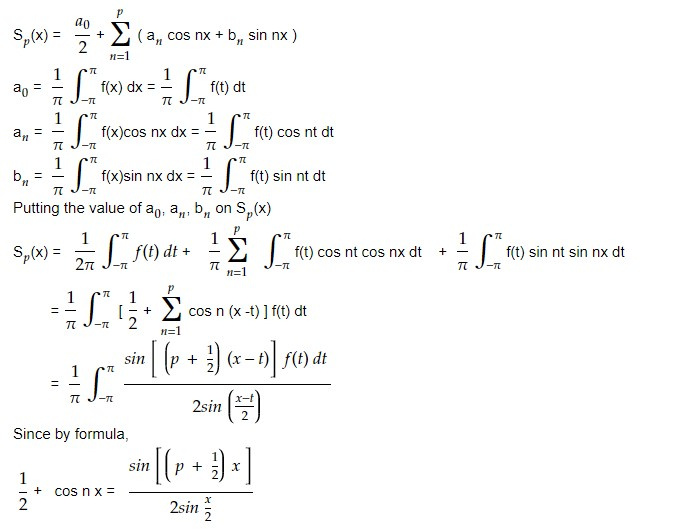As f(t) is periodic, we can shift the interval i.e we can change the limit as below:

Let θ = -(x-t)/2 ⇒ t = 2θ + x and dt = 2dθ

Also for t = - π +x

⇒ θ = - π/2

For t = π +x

⇒ θ = π/2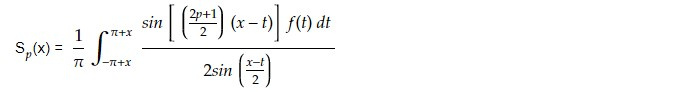Let θ = -(x-t)/2 ⇒ t = 2θ + x and dt = 2dθ

Also for t = - π +x

⇒ θ = - π/2

For t = π +x

⇒ θ = π/2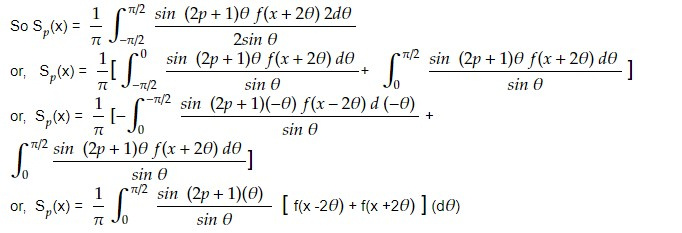For θ small sin θ ≃ θ. For p→ ∞ ⟹ (2p+1) → ∞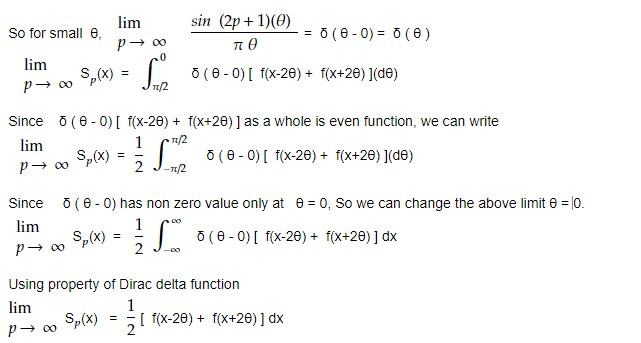At the value of x where f(x) is continuous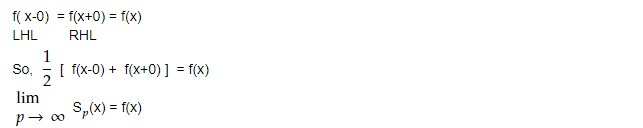And at the value of x where f(x) is discontinuous

f(x +0) ≠ f(x-0)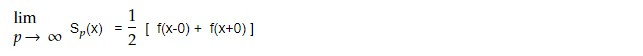This proves Dirichlet's theorem.

Gibb's phenomenon

Gibbs phenomenon is a phenomenon in signal processing and mathematical analysis where a truncated Fourier series approximation of a function exhibits oscillations near discontinuities. Specifically, if a Fourier series is used to approximate a function that has a discontinuity, such as a step function, the approximation will converge to the correct value at points away from the discontinuity, but near the discontinuity, the approximation will overshoot the correct value, leading to oscillations that do not converge to zero as the number of terms in the Fourier series is increased. These oscillations are known as Gibbs oscillations.

An example of Gibbs phenomenon is the reconstruction of a square wave from its samples. A square wave is a waveform that alternates abruptly between two levels. When a square wave is reconstructed from its samples, the reconstructed waveform has an overshoot that is proportional to the square root of the number of samples. This overshoot is known as Gibbs phenomenon.

For instance, consider a square wave with an amplitude of 1 and a period of 2π. When reconstructed using a finite number of samples, the reconstructed waveform will have an overshoot of approximately 8.8% of its amplitude at its peaks. This overshoot increases as the number of samples decreases, but the maximum overshoot is always 8.8% of the amplitude of the waveform.

The Gibbs phenomenon can be demonstrated by plotting the reconstructed waveform and comparing it to the original waveform. The overshoot can also be quantified by calculating the difference between the reconstructed waveform and the original waveform at its peaks.

This note is a part of the Physics Repository.